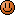# Expression Evaluation in Z80 Assembly

Tuesday, 24th February 2009

The expression evaluators I've written in the past have been memory hungry and complex. Reading the BBC BASIC ROM user's guide introduced me to the concept of expression evaluation using top-down analysis, which only uses a small amount of constant RAM and the stack.

I took some time out over the weekend to write an expression evaluator in Z80 assembly using this technique. It can take an expression in the form of a NUL-terminated string, like this:

```.db "(-8>>2)+ceil(pi())+200E-2**sqrt(abs((~(2&4)>>>(30^sin(rad(90))))-(10>?1)))",0
```

and produce a single answer (or an error!) in the form of a floating-point number. The source code and some notes can be downloaded here.

I initially wrote a simple evaluator using 32-bit integers. I supported the operations the 8-bit Z80 could do relatively easily (addition, subtraction, shifts and logical operations) and got as far as 32-bit multiplication before deciding to use BBC BASIC's floating-point maths package instead. The downside is that BBC BASIC has to be installed (the program searches for the application and calls its FPP routine).

I'm not sure if the technique used is obvious (I'd never thought of it) but it works well enough and the Z80 code should be easy to follow - someone may find it useful.